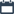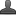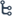Showing posts with label Multiplication of numbers with a series of 9's. Show all posts
Showing posts with label Multiplication of numbers with a series of 9's. Show all posts

Monday, November 16, 2020

•November 16, 2020
••, ,
•1 comment

Multiplication of numbers with a series of 9's,Facts,Quiz,more Maths topics

Multiplication of numbers with a series of 9's

Hi friends and my dear students! In this post, I have covered  Multiplication of numbers with a series of 9's  After Reading  Please do share it with your friends. Learn maths for All classes here

Multiplication of numbers with a series of 9's

In my seminars , I often have an audience challenge round . In this round , the audience members ask me to perform various mental calculations and give them the correct answer . They generally ask me to multiply numbers which involve a lot of 9's in them . The general perception is that the higher the number of 9's the tougher it will be for me to calculate . However , the truth is exactly the opposite - the higher the number of 9's in the question , the easier it is for me to calculate the correct answer . I use two methods for this . The first method is given below and the second method is explained in the chapter ' Base Method of Multiplication ' .

Using the method given below , we can multiply any given number with a series of nines . In other words , we can instantly multiply any number with 99 , 999,9999 , 99999 , etc.

The technique is divided into three cases . In the first case , we will be multiplying a given number with an equal number of nines . In the second case we will be multiplying a number with a higher number of nines . In the third case , we will be multiplying a number with a lower number of nines ,

Case 1 ( Multiplying a number with an equal number of nines )

( Q ) Multiply 654 by 999

• 654

x 999

-------

=653 346

• We subtract 1 from 654 and write half the answer as 653 . Answer at this stage is 653 . .

• Now we will be dealing with 653. Subtract each of the digits six , five and three from nine and write them in the answer one by one ,

•  Nine minus six is 3. Nine minus five is 4. Nine minus three is 6 .

• The answer already obtained was 653 and now we suffix to it the digits 3 , 4 and 6. The complete answer is 653346

Also Check

Vedic maths trick : Squaring of numbers between 50 and 60

( Q ) Multiply 9994 by 9999

• 9094

X9099

-----------

=9993 0006

We subtract one from 9994 and write it as 9993. This becomes our left half of the answer . Next , we . , 447 x 900 subtract each of the digits of 9993 from 9 and write the answer as 0006. This becomes the right half of the answer . The complete answer is 99930006

(Q) Multiply 456789 by 999999

•  156789

x 99999

------------------

=456789 543211

We subtract 1 from 456789 and get the answer 456788. We write this down on the left hand side . Next , we subtract each of the digits of 456788 ( left hand side ) from 9 and get 543211 which becomes the right hand part of our answer . The complete answer is 456788543211

More examples :

•  7777

X 9999

----------

=77762223

•  65432

X99999

-----------

=6543134568

• 447

X999

----------

=446553

• 90909

X99999

-----------

=9090809091

The simplicity of this method can be vouched from the examples given above . Now we move toCase 2. In this case , we will multiply a given number with a higher number of nines .

Case 2

( Multiplying a number with a higher number of nines )

( Q ) Multiply 45 with 999

45             045X999=044955

X 999

--------

There are three nines in the multiplier . However , the multiplicand 45 has only two digits . So we add a zero and convert 45 to 045 and make it a three digit number . After having done so , we can carry on with the procedure explained in Case 1

. First we subtract 1 from 045 and write it down as 044. Next , we subtract each of the digits of 044 from 9 and write the answer as 955. The complete answer is 044955 ,

( Q ) Multiply 888 with 9999

888             0888X9999 = 8879112

X9999

We convert 888 to 0888 and make the digits equal to the number of nines in the multiplier . Next , we subtract 1 from 0888 and write the answer as 0887. Finally , we subtract each digit of 0887 from 9 and write the answer as 9112. The final answer is 08879112 which is 8879112

( Q ) Multiply  123 by 99999

123              00123 X99999 =

x 99999          00122 / 99877

The multiplicand is a three - digit number and the multiplier is a five - digit number . Therefore  we

Also Check

Vedic maths trick :Squaring of numbers ending with ' 5

add two zeros in the multiplicand so that the digits are equal in the multiplicand and the multiplier .

We now subtract 1 from 00123 and write the left hand part of the answer as 00122. Next , we subtract each of the digits of the left hand part of the answer from 9 and write it down as 99877 as the right hand part of the answer . The complete answer is 12299877

Other examples :

•  162

X9999

-----------

0161/9838

•  5555

X99999

-----------

05554/94445

•  363

X999999

-------------

000362/999637

We can see that this technique is not only simple and easy to follow , but it also enables one to calculate the answer in the mind itself . This is the uniqueness of these systems . As you read the chapters of this book , you will realize how simple and easy it is to find the answer to virtually any problem of mathematics that one encounters in daily life and especially in the exams . And the approach is so different from the traditional methods of calculation that it makes the whole process enjoyable

Case 3 of this technique deals with multiplying a number with a lower number of nines . There is a separate technique for this in Vedic Mathematics and requires the knowledge of the Nikhilam Sutra ( explained later in this book ) . However , at this point of time , we can solve such problems using our normal practices of instant multiplication .

( Q ) Multiply 654 by 99 : In this case the number of digits are more than the number of nines in the multiplier . Instead of multiplying the number 654 with 99 we will multiply it with ( 100-1 ) . First we will multiply 654 with 100 and then we will subtract from it 654 multiplied by

1.

654

X 99

65400

-       654

--------------

= 64746

( Q ) Multiply 80020 by 999

We will multiply 80020 with ( 1000 - 1 ) .

80020000

-       800203

---------------

79939980

This method is so obvious that it needs no further elaboration .